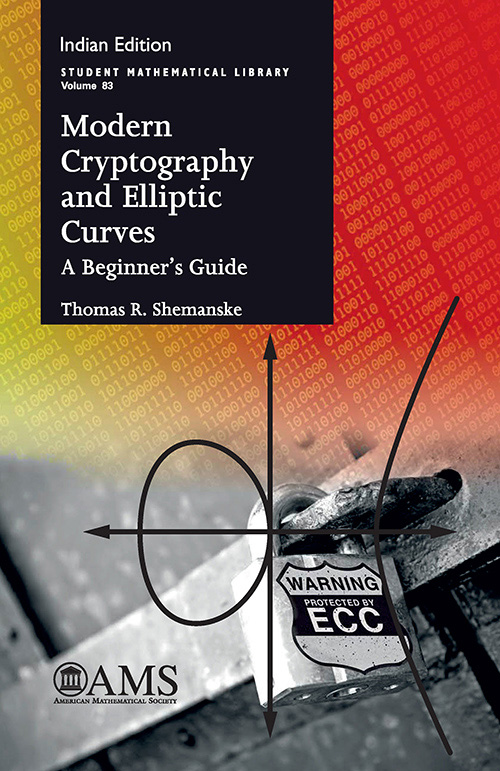## Modern Cryptography and Elliptic Curves

#### About the Book

This book offers the beginning undergraduate student some of the vista of modern mathematics by developing and presenting the tools needed to gain an understanding of the arithmetic of elliptic curves over finite fields and their applications to modern cryptography. This gradual introduction also makes a significant effort to teach students how to produce or discover a proof by presenting mathematics as an exploration, and at the same time, it provides the necessary mathematical underpinnings to investigate the practical and implementation side of elliptic curve cryptography (ECC).

Elements of abstract algebra, number theory, and affine and projective geometry are introduced and developed, and their interplay is exploited. Algebra and geometry combine to characterize congruent numbers via rational points on the unit circle, and group law for the set of points on an elliptic curve arises from geometric intuition provided by Bézout’s theorem as well as the construction of projective space. The structure of the unit group of the integers modulo a prime explains RSA encryption, Pollard’s method of factorization, Diffie–Hellman key exchange, and ElGamal encryption, while the group of points of an elliptic curve over a finite field motivates Lenstra’s elliptic curve factorization method and ECC.

The only real prerequisite for this book is a course on one-variable calculus; other necessary mathematical topics are introduced on-the-fly. Numerous exercises further guide the exploration.

#### Contributors (Author(s), Editor(s), Translator(s), Illustrator(s) etc.)

Thomas R. Shemanske, Dartmouth College, Hanover, NH

• Preface 8
Introduction 10

• Chapter 1. Three Motivating Problems 14
1.1. Fermat’s Last Theorem 16
1.2. The Congruent Number Problem 18
1.3. Cryptography 19

• Chapter 2. Back to the Beginning 22
2.1. The Unit Circle: Real vs. Rational Points 23
2.2. Parametrizing the Rational Points on the Unit Circle 25
2.3. Finding all Pythagorean Triples 29
2.4. Looking for Underlying Structure: Geometry vs. Algebra 40
2.5. More about Points on Curves 47
2.6. Gathering Some Insight about Plane Curves 51
2.7. Additional Exercises 56

• Chapter 3. Some Elementary Number Theory 58
3.1. The Integers 59
3.2. Some Basic Properties of the Integers 60
3.3. Euclid’s Algorithm 65
3.4. A First Pass at Modular Arithmetic 69
3.5. Elementary Cryptography: Caesar Cipher 76
3.6. Affine Ciphers and Linear Congruences 79
3.7. Systems of Congruences 83

• Chapter 4. A Second View of Modular Arithmetic: \Z_{n} and U_{n} 86
86
4.1. Groups and Rings 86
4.2. Fractions and the Notion of an Equivalence Relation 90
4.3. Modular Arithmetic 92
4.4. A Few More Comments on the Euler Totient Function 106
4.5. An Application to Factoring 108

• Chapter 5. Public-Key Cryptography and RSA 114
5.1. A Brief Overview of Cryptographic Systems 115
5.2. RSA 120
5.3. Hash Functions 127
5.4. Breaking Cryptosystems and Practical RSA Security Considerations 136

• Chapter 6. A Little More Algebra 140
6.1. Towards a Classification of Groups 141
6.2. Cayley Tables 141
6.3. A Couple of Non-abelian Groups 144
6.4. Cyclic Groups and Direct Products 147
6.5. Fundamental Theorem of Finite Abelian Groups 151
6.6. Primitive Roots 154
6.7. Diffie–Hellman Key Exchange 156
6.8. ElGamal Encryption 157

• Chapter 7. Curves in Affine and Projective Space 160
7.1. Affine and Projective Space 160
7.2. Curves in the Affine and Projective Plane 166
7.3. Rational Points on Curves 169
7.4. The Group Law for Points on an Elliptic Curve 172
7.5. A Formula for the Group Law on an Elliptic Curve 192
7.6. The Number of Points on an Elliptic Curve 198

• Chapter 8. Applications of Elliptic Curves 202
8.1. Elliptic Curves and Factoring 203
8.2. Elliptic Curves and Cryptography 209
8.3. Remarks on a Post-Quantum Cryptographic World 211

Appendix A. Deeper Results and Concluding Thoughts 216
A.1. The Congruent Number Problem and Tunnell’s Solution 216
A.2. A Digression on Functions of a Complex Variable 222
A.3. Return to the Birch and Swinnerton-Dyer Conjecture 224
A.4. Elliptic Curves over \C 225

Appendix B. Answers to Selected Exercises 232

Bibliography 258

Index 262

`